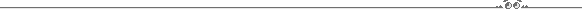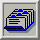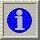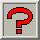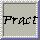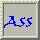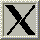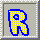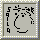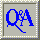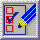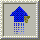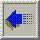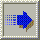##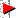3.2. Colour Vision

The human eye responds to long, middle and short wavelengths - these are red, green and blue. Normal visible light is a continuous spectrum starting at about 400 nanometers and going to about 700 nanometers - violet to red. There are many colour systems - the two most used are RGB and HSV. The RGB colour system is named after Red, Green and Blue. (The human eye reacts as though it has three sets of nerves corresponding to the red, green and blue parts of the visible spectrum.) Nearly every colour can be obtained by combining various amounts of red, green and blue. A different system - HSV - is named after the Hue, Saturation and Value of a colour (sometimes HSB Hue, Saturation and Brightness) and corresponds to the painter's tint, shade and tone.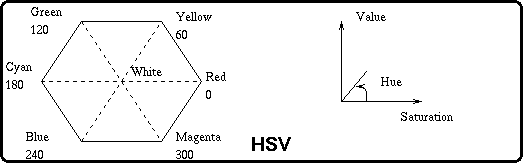The colour is obtained from the sides of the hexagon (shown above with full saturation and value), the amount of saturation of colour and the amount of white in the colour. The colour tuple is a point in the 3-space formed by the hexcone of the hexagon extruded and shrunk down to the point V=0 and S=0. Decreasing the Saturation means that more white is added, changing the Value means reducing the amount of the pure colour.

There are other colour systems - CIE (Commission Internationale de l'clairage), CMY (Cyan, Magenta and Yellow), YIQ, HLS (Hue, Lightness and Saturation), etc. Some are more relevant to image transmission (TV) than colour correctness.

In producing colour (Cathode Ray Tubes), we are using additive colour synthesis - white is produced by adding equal amounts of the three primaries. Colour on paper, etc is seen by subtractive colour synthesis - black is produced by mixing equal amounts of the primary dyes. As shown on the left hand side of

```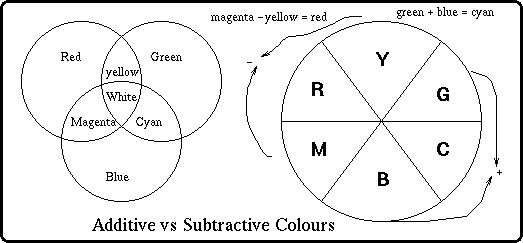Fig. 3.1 : Colour

```
Figure 3.1 , we can produce white by adding all three primaries.

To convert from RGB to CMY you use the following: C = 1 - R M = 1 - G Y = 1 - B
then convert to CMYK by K = min( C, M, Y ) C = C - K M = M - K Y = Y - K
Thus, going in the other direction, you'd just do R = 1 - ( C + K ) G = 1 - ( M + K ) B = 1 - ( Y + K )
Multiply the results by 255 and you're home free.

The code below shows how to convert from HSV to RGB.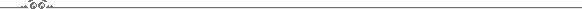```    #include <stdio.h>

/*
* Lifted straight from Foley, van Dam, Feiner, and Hughes, _Computer
* Graphics: Principles and Practice, Second Edition_.
*
* h varies between 0 and 360, s and v vary between 0 and 1.
* returns r, g, and b between 0 and 1.
*/

void hsv2rgb( double h, double s, double v, double *r, double *g, double *b )
{
if ( s == 0.0 )
{
if ( h < 0.0 )
{
*r = v; *g = v; *b = v;
}
else
{
fprintf( stderr, "hsv2rgb error -- invalid combination.\n" );
exit( 1 );
}
}
else
{
int i;
double f;
double p;
double q;
double t;

h = fmod( h, 360.0 );
if ( h < 0.0 )
h += 360.0;
h /= 60.0;
i = floor( h );
f = h - i;
p = v * ( 1.0 - s );
q = v * ( 1.0 - ( s * f ) );
t = v * ( 1.0 - ( s * ( 1.0 - f ) ) );
switch ( i )
{
case 0:
*r = v; *g = t; *b = p;
break;
case 1:
*r = q; *g = v; *b = p;
break;
case 2:
*r = p; *g = v; *b = t;
break;
case 3:
*r = p; *g = q; *b = v;
break;
case 4:
*r = t; *g = p; *b = v;
break;
case 5:
*r = v; *g = p; *b = q;
break;
default:
fprintf( stderr, "hsv2rgb error: Invalid case!  case = %d\n", i );
abort();

}
}
return;
}
```